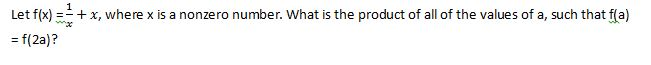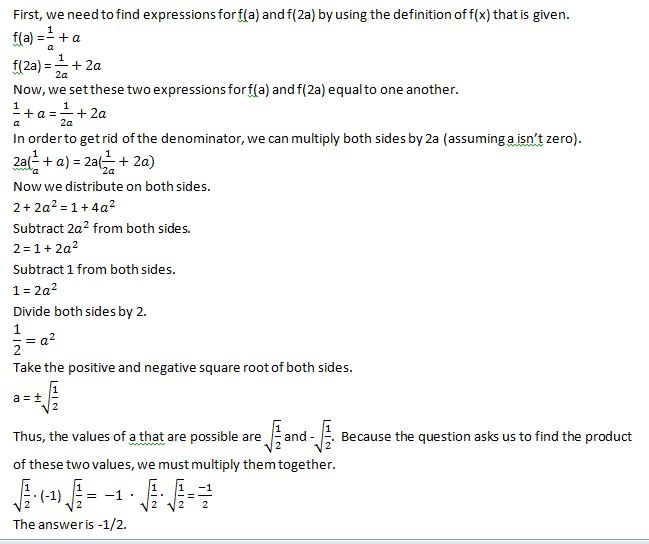Example Questions

Example Question #11 : Algebra

If y = 4 and 6y = 10z + y, then z = ?

2

24

4

6

30

2

Explanation:
1. Substitute y in the equation for 4.
2. You now have 6 * 4 = 10z + 4
3. Simplify the equation: 24 = 10z + 4
4. Subtract 4 from both sides: 24 – 4 = 10z + 4 – 4
5. You now have 20 = 10z
6. Divde both sides by 10 to solve for z.
7. z = 2.

Example Question #12 : Algebra

A sequence of numbers is: 2, 5, 8, 11. Assuming it follows the same pattern, what would be the value of the 20th number?

59

50

61

56

55

59

Explanation:

This goes up at a constant number between values, making it an arthmetic sequence. The first number is 2, with a difference of 3. Plugging this into the arithmetic equation you get A= 2 + 3 (n – 1). Plugging in 20 for n, you get a value of 59.

Example Question #13 : Algebra

The first four numbers of a sequence are 5, 10, 20, 40. Assuming the pattern continues, what is the 6th term of the sequence?

60

140

160

80

50

160

Explanation:

Looking at the sequence you can see that it doubles each term, making it a geometric sequence. Since it doubles r = 2 and the first term is 5. Plugging this into the geometric equation you get A= 5(2)n–1. Setting n = 6, you get 160 as the 6th term.

Example Question #14 : Algebra

Given f(x) = x– 9. What are the zeroes of the function?

0, 3

0

3

–3, 0, 3

–3, 3

–3, 3

Explanation:

The zeroes of the equation are where f(x) = 0 (aka x-intercepts). Setting the equation equal to zero you get x2 = 9. Since a square makes a negative number positive, x can be equal to 3 or –3.

Example Question #15 : Algebra

Give the lines y = 0.5x+3 and y=3x-2. What is the y value of the point of intersection?

4

6

3

2

7

4

Explanation:

In order to solve for the x value you set both equations equal to each other (0.5x+3=3x-2). This gives you the x value for the point of intersection at x=2. Plugging x=2 into either equation gives you y=4.

Example Question #16 : Algebra

10 gallons of paint will cover 75 ft2. How many gallons of paint will be required to paint the area of a rectangular wall that has a height of 8 ft and a length of 24 ft?

25.6 gallons

22.8 gallons

14.2 gallons

17 gallons

31.4 gallons

25.6 gallons

Explanation:

First we need the area or the rectangle. 24 * 8 = 192. So now we know that 10 gallons will cover 75 ft2 and x gallons will cover 192 ft2. We set up a simple ratio and cross multiply to find that 75x = 1920.

x = 25.6

Example Question #17 : Algebra

What number decreased by 7 is equal to 10 increased by 7?

15

27

24

17

10

24

Explanation:

The best way to solve this problem is to translate it into an equation, "decreased" meaning subtract and "increased" meaning add:

x – 7 = 10 + 7

x = 24

Example Question #18 : Algebra

If a%b = (2b + 3a)/(6ab), what would have a greater value, 2%3 or 3%2?

2%3

3%2

Cannot be determined

They are the same

3%2

Explanation:

First find 2%3 = (2 * 3 + 3 * 2)/(6 * 2 * 3) = 12/36 = 1/3, then 3%2 = (2 * 2 + 3 * 3)/(6 * 3 * 2) = 13/36 which is greater.

Example Question #19 : Algebra–1

0

1/2

1

–1/2

–1/2

Explanation:Example Question #20 : Algebra

If 5 + x is 5 more than 5,what is the value of 2x?

15

5

10

20